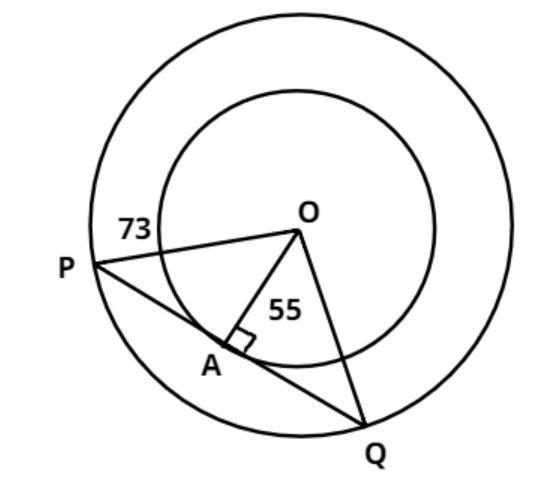Courses
Courses for Kids
Free study material
Free LIVE classes
MoreLIVE
Join Vedantu’s FREE Mastercalss

# Two concentric circles of radii 73cm and 55cm. find the length of the chord of the larger circle which touches the smaller circle.Verified
364.2k+ views
Hint: - Perpendicular drawn from the center to the chord of the circle bisects the chord.Two concentric circles of radii 55cm and 73cm are shown in figure.
$\Rightarrow {\text{OA = 55cm and OP = 73cm}}$
Now we have to find out the chord of the larger circle which touches the smaller circle.
$\Rightarrow$So, from the figure we have to find out the value of PQ.
Since OA is perpendicular on the chord PQ, therefore triangle OAP is a right angle triangle at A
So, apply Pythagoras Theorem in triangle OAP, we have
${\text{O}}{{\text{A}}^2}{\text{ + A}}{{\text{P}}^2}{\text{ = O}}{{\text{P}}^2} \\ \Rightarrow {\text{A}}{{\text{P}}^2} = {73^2} - {55^2} \\ \Rightarrow {\text{A}}{{\text{P}}^2} = 5329 - 3025 = 2304 \\ \Rightarrow {\text{AP = 48cm}} \\$
As we know that the perpendicular drawn from the center to the chord of the circle bisects the chord.
$\therefore {\text{PQ = 2AP = 2}} \times 48 = 96cm$
So, the length of the chord which touches the smaller circle is 96 cm.

Note: - Concentric circles are the circles with a common center and whenever we face such types of problem first draw the pictorial representation then draw the perpendicular from the center on the chord of the circle which divide the chord into two equal parts, then apply Pythagoras Theorem we will get the required answer.
Last updated date: 23rd Sep 2023
Total views: 364.2k
Views today: 10.64k